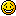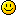# Sorry your browser is not supported!

You are using an outdated browser that does not support modern web technologies, in order to use this site please update to a new browser.

Browsers supported include Chrome, FireFox, Safari, Opera, Internet Explorer 10+ or Microsoft Edge.

### FPSC Classic Scripts / In case if you wanna change 'weapon pickup' title into game

Message
Posted: 23rd Apr 2018 21:48
Hello guyz! I wanna share something that helps you change some game facilities in yo projectHere is an open-source cod I've found in FPSC directory that you can find there "(Disc name):/Program Files/The Game Creators/FPS Creator/Files (files folder)/scriptbank/weaponglow"
Seen right in this video

*****script*****

You can change thy in case of number range of keynumbers. I hope it will solve your technical problems and let you 'play with settings'*****scancodekeys*****

01 = Escape
02 = 1 or !
03 = 2 or @
04 = 3 or #
05 = 4 or \$
06 = 5 or %
07 = 6 or ^
08 = 7 or &
09 = 8 or *
0A = 9 or (
0B = 0 or )
0C = - or _
0D = = or +
0E = Backspace
0F = Tab
10 = q or Q
11 = w or W
12 = e or E
13 = r or R
14 = t or T
15 = y or Y
16 = u or U
17 = i or I
18 = o or O
19 = p or P
1A = [ or {
1B = ] or }
1C = Enter
1D = Control
1E = a or A
1F = s or S
20 = d or D
21 = f or F
22 = g or G
23 = h or H
24 = j or J
25 = k or K
26 = l or L
27 = ; or :
28 = ' or "
29 = ` or ~
2A = Left shift
2B = \ or |
2C = z or Z
2D = x or X
2E = c or C
2F = v or V
30 = b or B
31 = n or N
32 = m or M
33 = ,
34 = .
35 = / or ?
36 = Right shift
37 = * or PrtScr
38 = Alt key
39 = Space bar
3A = Caps Lock
3B = F1
3C = F2
3D = F3
3E = F4
3F = F5
40 = F6
41 = F7
42 = F8
43 = F9
44 = F10
45 = Num Lock on numeric keypad
46 = Scroll Lock on numeric keypad
47 = 7 or Home on numeric keypad
48 = 8 or Cursor Up on numeric keypad
49 = 9 or Pg Up on numeric keypad
4A = - on numeric keypad
4B = 4 or Cursor Left on numeric keypad
4C = 5 key on numeric keypad
4D = 6 or Cursor Right on numeric keypad
4E = + key on numeric keypad
4F = 1 or End on numeric keypad
50 = 2 or Cursor Down on numeric keypad
51 = 3 or Pg Dn on numeric keypad
52 = 0 or Insert on numeric keypad
53 = . or Delete on numeric keypad
54 = Sys Req
57 = F11
58 = F12
E1 = Pause
1C = Enter on numeric keypad
1D = Control
35 = / on numeric keypad
38 = Alt
47 = Home
48 = Up arrow
49 = Pg Up
4B = Left arrow
4D = Right arrow
4F = End
50 = Down arrow
51 = Pg Dn
52 = Insert
53 = Delete
Posted: 28th Apr 2018 17:05
@ kir0s Yes, very easy to do when one knows howThanks
My games never have bugs. They just develop random features..
Lots and lots of random features...